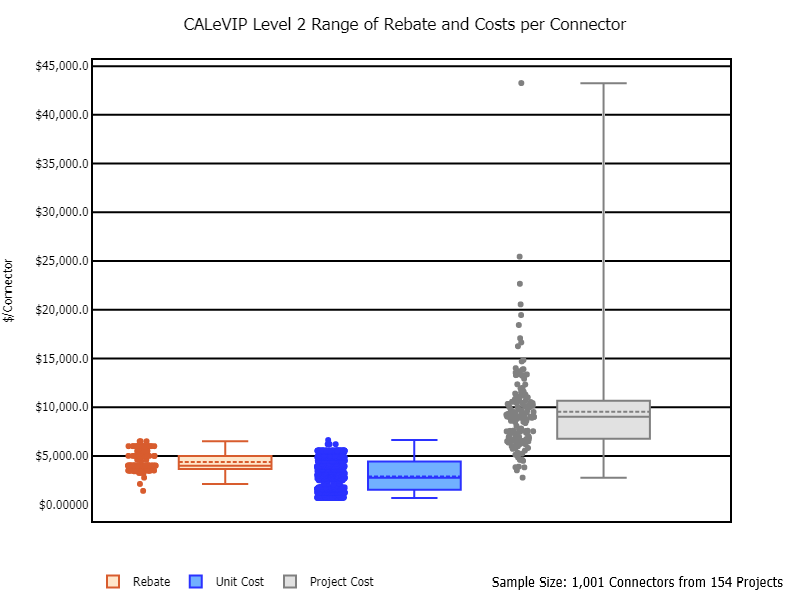The box plots show the range of rebate per connector, unit cost per connector, and total project cost per connector for level 2 CALeVIP projects.

The rebate per connector ranged from a minimum of \$1,410 to a maximum of \$6,500 with a mean and median of \$4,384 and \$4,000, respectively. The unit cost per connector ranged from a minimum of \$685 to a maximum of \$6,626 with a mean and median of \$2,896 and \$2,748, respectively. The total project cost per connector ranged from a minimum of \$2,769 to a maximum of \$43,264 with a mean and median of \$9,540 and \$9,014, respectively.

Each point shown next to the respective box plot represents the rebate, equipment cost, and total project cost for one project. The top and bottom ends of the box are the upper and lower quartiles, respectively. The box spans the interquartile range. The horizontal line within the box represents the median value. The horizontal dotted line within the box represents the mean value. The whiskers are the two lines that extend above and below the box and represent the highest and lowest values. This graph excludes sites where the site host installed both Level 2 and DC Fast Chargers under a single rebate.

## Contact

Fuels and Transportation Division
FTD@energy.ca.gov